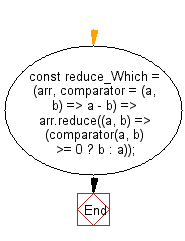# JavaScript: Return the minimum-maximum value of an array, after applying the provided function to set comparing rule

## JavaScript fundamental (ES6 Syntax): Exercise-15 with Solution

Write a JavaScript program to return the minimum-maximum value of an array, after applying the provided function to set comparing rule.

• Use Array.prototype.reduce() in combination with the comparator function to get the appropriate element in the array.
• Omit the second argument, comparator, to use the default one that returns the minimum element in the array.

Sample Solution:

JavaScript Code:

``````//#Source https://bit.ly/2neWfJ2
const reduce_Which = (arr, comparator = (a, b) => a - b) =>
arr.reduce((a, b) => (comparator(a, b) >= 0 ? b : a));
console.log(reduce_Which([1, 3, 2]));
console.log(reduce_Which([10, 30, 20], (a, b) => b - a));
console.log(reduce_Which(
[{ name: 'Kevin', age: 16 }, { name: 'John', age: 20 }, { name: 'Ani', age: 19 }],
(a, b) => a.age - b.age));
```
```

Sample Output:

```1
30
{"name":"Kevin","age":16}
```

Flowchart:Live Demo:

See the Pen javascript-basic-exercise-1-15 by w3resource (@w3resource) on CodePen.

Improve this sample solution and post your code through Disqus

What is the difficulty level of this exercise?

Test your Programming skills with w3resource's quiz.

﻿

## JavaScript: Tips of the Day

Returns an array of n-tuples of consecutive elements

Example:

```const tips_arr = (n, arr) =>  n > arr.length ? [] : arr.slice(n - 1).map((v, i) =>[...arr.slice(i, i + n - 1), v]);
console.log(tips_arr(2, [1, 2, 3, 4, 5]));
console.log(tips_arr(3, [1, 2, 3, 4, 5]));
console.log(tips_arr(5, [1, 2, 3, 4]));
```

Output:

```[[1, 2], [2, 3], [3, 4], [4, 5]]
[[1, 2, 3], [2, 3, 4], [3, 4, 5]]
[]
```